# RS Aggarwal Class 7 Solutions Chapter 20 Mensuration Ex 20E

## RS Aggarwal Class 7 Solutions Chapter 20 Mensuration Ex 20E

These Solutions are part of RS Aggarwal Solutions Class 7. Here we have given RS Aggarwal Solutions Class 7 Chapter 20 Mensuration Ex 20E.

Other Exercises

Question 1.
Solution:
Radius of the circle (r) = 28 cm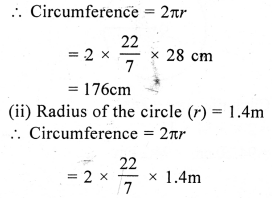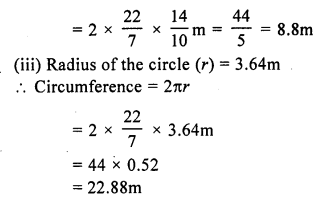Question 2.
Solution:
(i) Diameter of circle (d) = 35 cm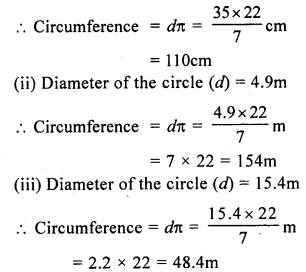Question 3.
Solution:
Radius of a circle = 15 cm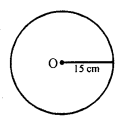Circumference = 2πr = 2 x 3.14 x 15 = 94.20 cm = 94.2 cm

Question 4.
Solution:
Circumference of a circle (c) = 57.2 cm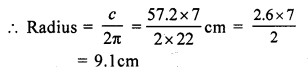Question 5.
Solution:
Circumference (c) = 63.8 m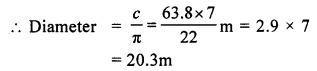Question 6.
Solution:
Let c be the circumference and d be the diameter of the circle.
c = d + 30
⇒ dπ = d + 30
⇒ dπ – d = 30
⇒ d (π – 1) = 30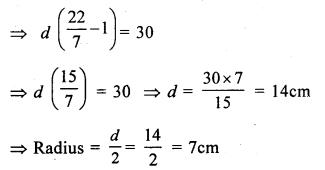Question 7.
Solution:
The ratio of the radii of the circles = 5 : 3
Let radius of first circle = 5x
and radius of second circle = 3x
Circumference of first circle = 2πr = 2π x 5x = 10πx
and circumference of second circle = 2π x 3x = 6πx
Ratio = 10πx : 6πx = 10 : 6 = 5 : 3

Question 8.
Solution:
Radius of circular field (r) = 21 m
Circumference = 2πr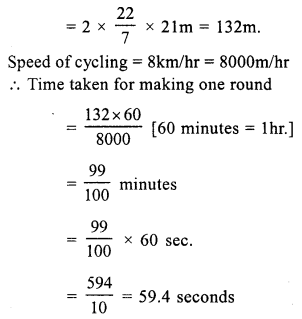Question 9.
Solution:
Outer circumference = 616 m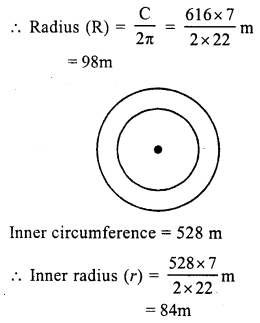Width of track = R – r = 98 – 84 = 14m

Question 10.
Solution:
Inner circumference of the circular track = 330 m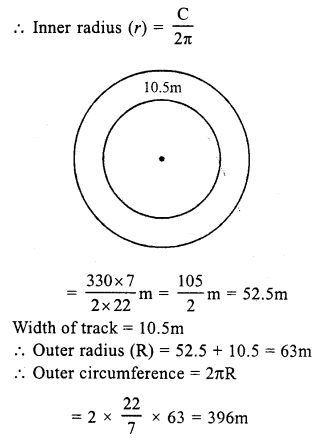Rate of fencing = Rs. 20 per metre
Total cost = Rs. 20 x 396 = Rs. 7920

Question 11.
Solution:
Radius of inner circle (r) = 98 cm
Inner circumference = 2πr = 2 x $$\frac { 22 }{ 7 }$$ x 98 cm = 616 cm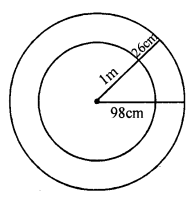Radius of the outer circle (R) = 1 m 26cm = 126 cm
Outer circumference = 2πR = 2 x $$\frac { 22 }{ 7 }$$ x 126 cm = 792 cm

Question 12.
Solution:
Side of equilateral triangle = 8.8 cm
Its perimeter = 8.8 x 3 = 26.4 cm
By bending their wire into a circular shape,
the circumference = 26.4 cm
Let d be the diameter,
Then C = dπ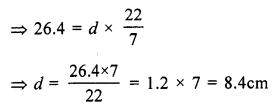Question 13.
Solution:
Each side of rhombus = 33 cm
Perimeter = 4 x 33 = 132 cm
Perimeter of circle = 132 cm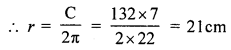Question 14.
Solution:
Length of rectangle (l) = 18.7 cm
Perimeter = 2 (l + b) = 2 (18.7 + 14.3) cm = 2 x 33 = 66 cm
Circumference of the so formed circle = 66 cm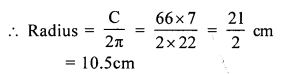Question 15.
Solution:
Radius of the circle (r) = 35 cm
Its circumference (c) = 2πr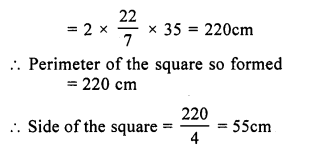Question 16.
Solution:
Diameter of well (d) = 140 cm
Outer circumference of parapet = 616 cm
Let D be the diameter, then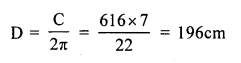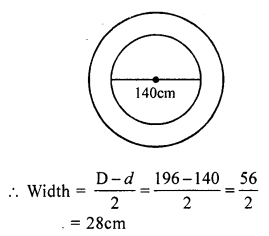Question 17.
Solution:
Diameter of wheel (d) = 98 cm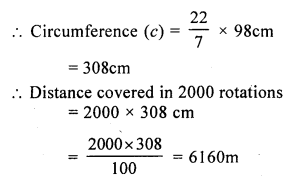Question 18.
Solution:
Diameter of cycle wheel (d) = 70 cm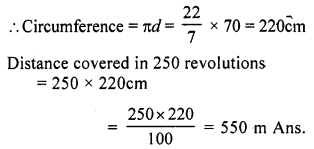Question 19.
Solution:
Diameter of car wheel (d) = 77 cm
Circumference = πd = $$\frac { 22 }{ 7 }$$ x 77 cm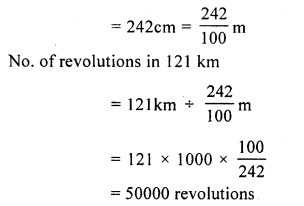Question 20.
Solution:
No. of revolutions = 5000
Distance covered = 11 km = 11 x 1000 = 11000 m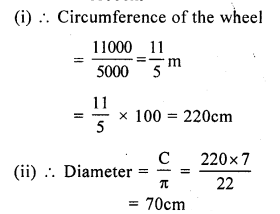Question 21.
Solution:
Length of hour hand (r) = 4.2 cm
and length of minutes hand (R) = 7cm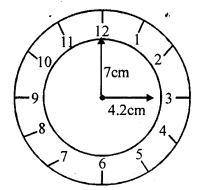Distance covered by hour hand in 24 hours = 2πR
= 2 x $$\frac { 22 }{ 7 }$$ x 4.2
= 26.4 cm
But distance covered by minute hand in one hour = 2πR = 2 x $$\frac { 22 }{ 7 }$$ x 7 = 44 cm
and distance covered by minute hand in 24 hours = 44 x 24 cm = 1056 cm
Sum of distance covered by these hands = 26.4 + 1056 = 1082.4 cm

Hope given RS Aggarwal Solutions Class 7 Chapter 20 Mensuration Ex 20E are helpful to complete your math homework.

If you have any doubts, please comment below. Learn Insta try to provide online math tutoring for you.